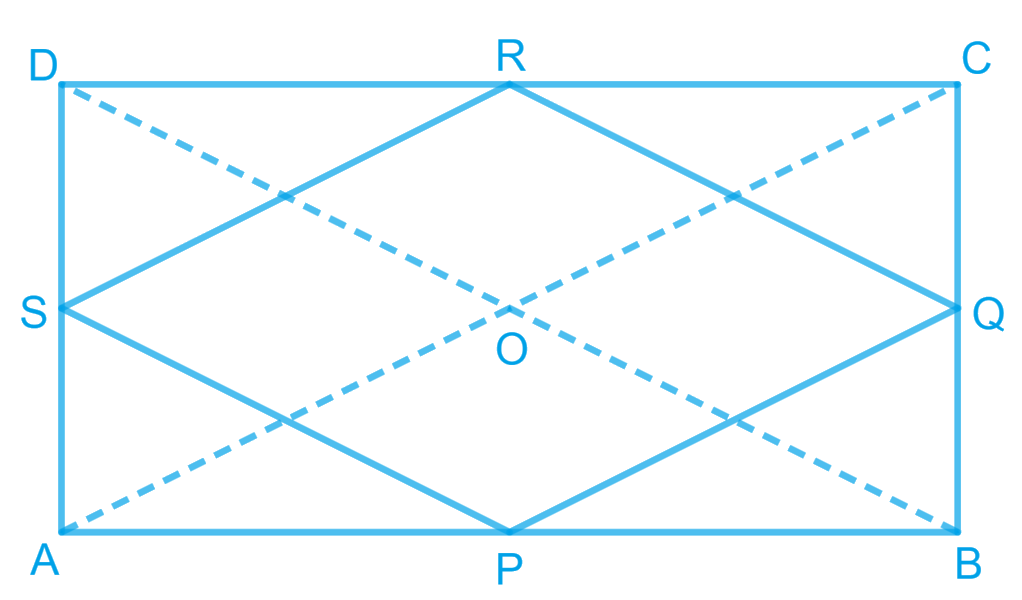# Ex.8.2 Q3 Quadrilaterals Solution - NCERT Maths Class 9

Go back to  'Ex.8.2'

## Question

$$ABCD$$ is a rectangle and $$P$$, $$Q$$, $$R$$ and $$S$$ are mid-points of the sides $$AB$$, $$BC$$, $$CD$$ and $$DA$$ respectively. Show that the quadrilateral $$PQRS$$ is a rhombus.

Video Solution
Ex 8.2 | Question 3

## Text Solution

What is known?

$$ABCD$$ is a rectangle and $$P, Q, R$$ and $$S$$ are the mid-points of the sides$$AB, BC, CD\,and\, DA$$ respectively.

What is unknown?

How we can show that quadrilateral $$PQRS$$ is a rhombus.

Reasoning:

In a triangle, the line segment joining the mid-points of any two sides of the triangle is parallel to the third side and is half of it. Also if one pair of opposite sides of quadrilateral is parallel and equal to each other then it is a parallelogram. By showing all four sides equal in parallelogram we can say it is rhombus.

Steps:Let us join $$AC$$ and $$BD$$.

In $$\Delta$$$$ABC$$,

$$P$$ and $$Q$$ are the mid-points of $$AB$$ and $$BC$$ respectively.

\begin{align}&\therefore PQ \parallel AC \; and \; PQ =\frac{1}{2} \\&AC \text{(Mid-point theorem)} ... (1)\end{align}

Similarly, in $$\Delta \text{ADC,}$$

\begin{align}& SR \parallel AC \; and \; SR =\frac{1}{2}\\& AC \text{(Mid-point theorem)} ... (2)\end{align}

Clearly, \begin{align} PQ \parallel SR \; and \; PQ = SR \end{align}

Since in quadrilateral $$PQRS$$, one pair of opposite sides is equal and parallel to each other, it is a parallelogram.

\begin{align}&\therefore \,\,{{PS}}\,\,{{||}}\,\,{{QR}}\,{{and}}\,{{PS}}\,\,{{ = }}\,{{QR}}\,\\&\left( \begin{array}{l}{\text{Opposite sides }}\\{\text{of parallelogram}}\end{array} \right)\, \ldots \left( 3 \right)\end{align}

In $$\Delta$$ $$BCD$$, $$Q$$ and $$R$$ are the mid-points of side $$BC$$ and $$CD$$ respectively.

\begin{align}& \therefore QR \parallel BD \; and \; QR =\frac{1}{2}\\& BD\text{ (Mid-point theorem)} ... (4)\end{align}

However, the diagonals of a rectangle are equal.

$$\therefore$$  $$AC = BD$$... (5)

By using Equations ($$1$$), ($$2$$), ($$3$$), ($$4$$), and ($$5$$), we obtain

$$PQ= QR= SR= PS$$

Therefore, $$PQRS$$ is a rhombus.

Video Solution